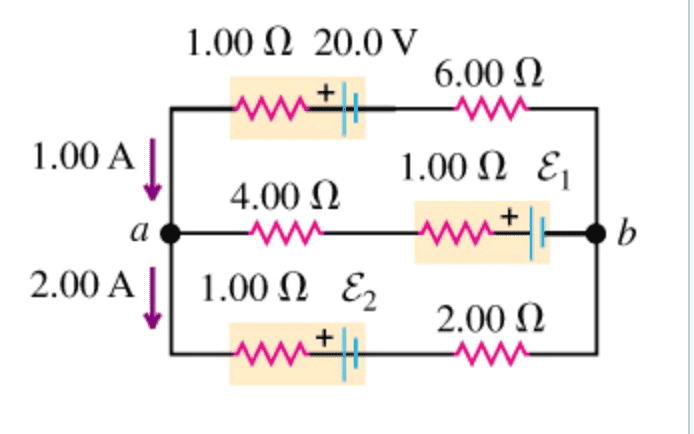# Potential difference across two points in a circuit

## Homework Statement

[/B]
Find the potential difference between points b and a in the circuit belowI have already solved for the voltages of the two batteries (1 and 2) in the circuit (18 V and 7 V respectively)

2. Homework Equations

Kirchhoff's Rules
1) Potential difference across any closed loop is 0
2) Current in = current out

## The Attempt at a Solution

[/B]
I have read that Kirchhoff's current rule is how to approach these kinds of problems, but I am not sure how.

ehild
Homework Helper
You have found the voltages of the batteries correctly. How did you do it? For that, you had to use the potential difference between points a and b, that you know from the uppermost branch of the circuit.

I used Kirchhoffs voltage law to find the battery voltages. Could you elaborate on how I unknowingly found the potential difference between b and a please?

ehild
Homework Helper
I used Kirchhoffs voltage law to find the battery voltages. Could you elaborate on how I unknowingly found the potential difference between b and a please?

For battery 2:

-2(1) - E2 - 2(2) - 1(6) + 20 -1(1) = 0, solve for E2 (Kirchhoff's voltage law around the large loop)

For battery 1:

E1 - 1(1) - 1(4) - 2(1) - E2 - 2(2) = 0, solve for E1 (Kirchhoff's voltage law around the bottom half loop)

ehild
Homework Helper
For battery 2:

-2(1) - E2 - 2(2) - 1(6) + 20 -1(1) = 0, solve for E2 (Kirchhoff's voltage law around the large loop)

You started from point a and and followed the change of potential along the current. The potential change from a to b is Ub-Ua = -2(1) - E2 - 2(2) .
From b to a, to potential changes by - 1(6) + 20 -1(1)= Ua-Ub. The potential difference between b and a is Ub-Ua = ??? :)

You started from point a and and followed the change of potential along the current. The potential change from a to b is Ub-Ua = -2(1) - E2 - 2(2) .
From b to a, to potential changes by - 1(6) + 20 -1(1)= Ua-Ub. The potential difference between b and a is Ub-Ua = ??? :)

Oh, I think I understand. So if I start at point b and end at point a:

-1(6) + 20 - 1(1) = Vb - Va = 13 V

Is this correct? I only I have one try left, so I don't want to squander it.

ehild
Homework Helper
It must be correct.

Ok, I got the magnitude right (13) but the sign wrong (it was - 13 V). I don't understand why. If it wanted the potential difference from b to a, why would you not start at b and work your way towards a?

ehild
Homework Helper
It depends how the potential difference between points a and b is defined. Usually it is Ua-Ub. But the question was the potential between b and a, which is Ub-Ua, and it is negative.

Could you explain how to get the sign right? I don't understand why it's negative rather than positive.

ehild
Homework Helper
Could you explain how to get the sign right? I don't understand why it's negative rather than positive.

In Post #6, the potential changed from Vb to Va by 13 V. So Va =13+ Vb, Va-Vb =13 . When it is said "potential difference between X and Y" your book means that you have to take the change of potential from Y to X: VX-VY.
The question was
Find the potential difference between points b and a in the circuit below

You have to find the potential at b with respect to a, that is, Vb-Va. Vb-Va = - (Va-Vb) = -13 V